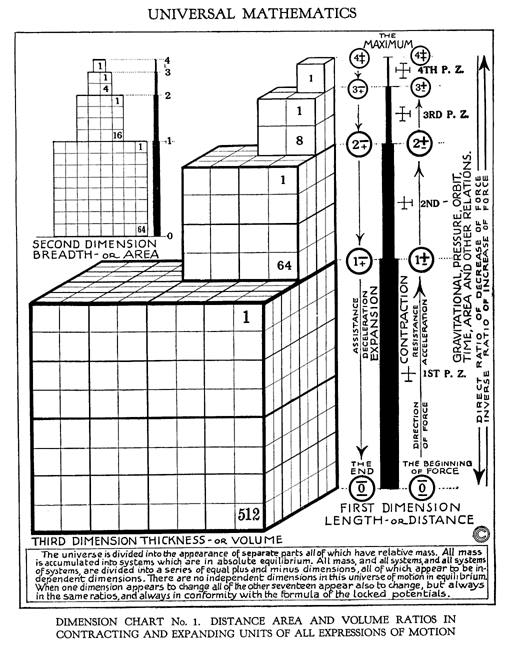# Table 12.02 - Length Area and Volume MathFigure 12.09 - Dimensions and Their Relationships

In graphic Figure 12.09 - Dimensions and Relationships it is clear:

Relative Volume

Accumulating Dispersing

4+ = 1/8 of 3+ or 3+ = 8 X 4+ or 81

3+ = 1/8 of 2+ or 2+ = 8 X 3+ or 82

2+ = 1/8 of 1+ or 1+ = 8 X 2+ or 83

Numeric Progressions (units)

1st Dimension = Linear = 1, 2, 4, 8.. (Doubling, nX2)

2nd Dimension = Area = 1, 4, 8, 64.. (Squaring, n2)

3rd Dimension = Volume = 1, 8, 64, 512.. (Cubing, n3)

Volumes

Cube Volume = 1 = 13

Cube Volume = 2 = cube root of 2 = 1.259922 on side

Cube Volume = 4 = cube root of 4 = 1.587403 on side

Cube Volume = 8 = cube root of 8 = 2 on side

therefore

Wavelengths and Frequencies - Octave Relations of Russell's Indig Number System

 Indig Vol. Units Vol. Calc Wavelength Example Octave Note 4 1 13 1 1 cps 4 G as 4th octave 3 8 23 2 1/2 cps 3 F as 3rd octave 2 64 43 4 1/4 cps 2 E as 2nd octave 1 512 83 8 1/8 ccps 1 D as 1st octave 0 C## non-octave

Table 12.02.01 - Wavelengths and Frequencies

Showing linear versus geometric progressions as also other types of progressions (counting methods or scales).

arithmetical progression
Frequency
Geometrical Progression
Laws of Being
progression
Ratio
Reciprocal
Reciprocating Proportionality
Square Law
Table 12.02 - Length Area and Volume Math
Tone
Volume
wave number
Wavelength
12.00 - Reciprocating Proportionality
12.18 - Multiple Octave Progression

References
Calculate various Properties of a Cylinder

Area
Cube
Length
Sphere
Volume